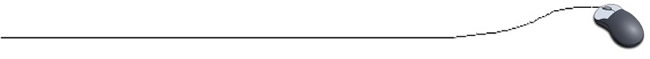Searching for a Specific Value

***Definition:  A key is a value that you are looking for in an array.

The simplest type of search is the sequential search (or linear search).  In the sequential search, each element of the array is compared to the key, in the order it appears in the array, until the desired element is found.  If you are looking for an element that is near the front of the array, the sequential search will find it quickly.  The more data that must be searched, the longer it will take to find the data that matches the key.

Consider this method which will search for a key integer value.  If found, the index (subscript) of the first location of the key will be returned.  If not found, a value of -1 will be returned.

 public static int search(int [ ] numbers, int key) {    for (int index = 0; index < numbers.length; index++)     {            if ( numbers[index] = = key )                  return index;  //We found it!!!     }   // If we get to end of loop, a value has not yet   // been returned.  The key in not in this array.    return -1; } If the key value is found, the index (subscript) of the location is returned.  This tells us that the return value x, will be the first integer found such that numbers [ x ] = key. There may be additional key locations in this array beyond this location.Now, suppose you are searching for  the number of times a specific key value is included in an array:

//Find the number of times the name "Jones" appears in an array of names

public static void main(String[] args)
{
String key = "Jones"; //case sensitive - "Jones" will not equal "jones"
String[ ] list = new String ;   // instantiate the array
for ( int i=0; i<100; i++)     // fill the array from the keyboard
{
System.out.println("Enter name: ");
}
int count = search (list, key);    // invoke the method
System.out.println("Count = " + count);
}
public static int search(String [ ] list, String key) //method to find "Jones"
{
int i, count = 0;
for( i = 0; i< list.length; i++)
{
if (list [ i ].equals( key ))
count = count+1;
}
return (count);

Here is yet another manner of searching:

 Searching with BREAK and boolean: // Search for the number 31 in a set of integers // This search takes place in main. // This search could also have been placed in a method. public class BreakBooleanDemo {      public static void main(String[ ] args)      {            int[ ] numbers = { 12, 13, 2, 33, 23, 31, 22, 6, 87, 16 };            int key = 31;            int i = 0;            boolean found = false;    // set boolean value to false until key is found           for ( i = 0; i < numbers.length; i++)           {                  if (numbers[ i ]  == key)                 {                          found = true;                               break;                  }            }           if (found)   //When found is true, index of location of key is printed.           {                 System.out.println("Found " + key + " at index " + i + ".");           }           else           {                 System.out.println(key + "is not in this array.");           }       } } OUTPUT: Found 31 at index 5.